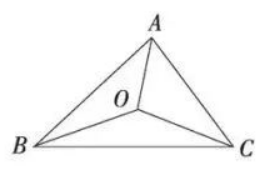$\text{A.}$ 20 $\text{B.}$ 15 $\text{C.}$ 18 $\text{D.}$ 12

B